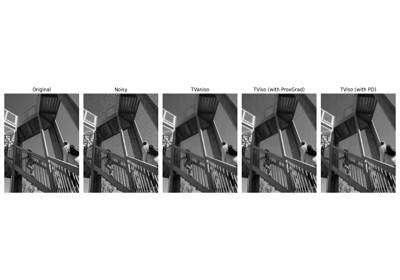pyproximal.optimization.primal.LinearizedADMM(proxf, proxg, A, x0, tau, mu, niter=10, callback=None, show=False)[source]#

Linearized Alternating Direction Method of Multipliers

Solves the following minimization problem using Linearized Alternating Direction Method of Multipliers (also known as Douglas-Rachford splitting):

$\mathbf{x} = \argmin_\mathbf{x} f(\mathbf{x}) + g(\mathbf{A}\mathbf{x})$

where $$f(\mathbf{x})$$ and $$g(\mathbf{x})$$ are any convex function that has a known proximal operator and $$\mathbf{A}$$ is a linear operator.

Parameters
proxfpyproximal.ProxOperator

Proximal operator of f function

proxgpyproximal.ProxOperator

Proximal operator of g function

Apylops.LinearOperator

Linear operator

x0numpy.ndarray

Initial vector

taufloat, optional

Positive scalar weight, which should satisfy the following condition to guarantee convergence: $$\mu \in (0, \tau/\lambda_{max}(\mathbf{A}^H\mathbf{A})]$$.

mufloat, optional

Second positive scalar weight, which should satisfy the following condition to guarantees convergence: $$\mu \in (0, \tau/\lambda_{max}(\mathbf{A}^H\mathbf{A})]$$.

niterint, optional

Number of iterations of iterative scheme

callbackcallable, optional

Function with signature (callback(x)) to call after each iteration where x is the current model vector

showbool, optional

Display iterations log

Returns
xnumpy.ndarray

Inverted model

znumpy.ndarray

Inverted second model

ADMM

ADMML2

Notes

The Linearized-ADMM algorithm can be expressed by the following recursion:

$\begin{split}\mathbf{x}^{k+1} = \prox_{\mu f}(\mathbf{x}^{k} - \frac{\mu}{\tau} \mathbf{A}^H(\mathbf{A} \mathbf{x}^k - \mathbf{z}^k + \mathbf{u}^k))\\ \mathbf{z}^{k+1} = \prox_{\tau g}(\mathbf{A} \mathbf{x}^{k+1} + \mathbf{u}^k)\\ \mathbf{u}^{k+1} = \mathbf{u}^{k} + \mathbf{A}\mathbf{x}^{k+1} - \mathbf{z}^{k+1}\end{split}$

## Examples using pyproximal.optimization.primal.LinearizedADMM#Denoising

Denoising